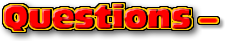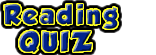ChaptersClick for the questions that go with this reading
Solving by ratios

Solving by rations involves very few measured quantities. These questions have phrases in them that describe factors of change. The will describe factors of change by saying a quantity doubles or triples. It might say that a quantity changes by a fraction factor, e.g. "...the radius changes by a factor of 5/8's."

The process
1. Start by list the given to a problem and the factors of change.
2. Then search for a formula or formula that contain these variables and the unknown.
3. You are going to make a big mixed fraction. In the denominator, write the equation with only variables.
4. In the numerator, write the equation with the factors of change.
5. Divide on equation by the other. in other words, any variable or number that appears in the numerator's equation is deleted.
6. A new math equation will be generated. Use this to solve for the factor of change. See the examples below.

 Math note: When you are dealing with a variable that is raised to a power, whatever happens to the variable also happens to the factor of change. Example: Suppose in a problem the velocity is tripled the formula you need to use has a v2 in it. In the numerator's formula, v2 becomes (3v)2 this expands to 32 v2 . When the v2 is divided out in the next step notice that the 32 remains squared. See the examples below to see how this happens.

Example #3
• Question
• Solution
• Video Solution
A car is traveling around two curves. On one curve the car is traveling around triple the speed of the first but the second curve has 2 times the radius of the first. How does the centripetal force compare between the two curves.This video can be found on YouTube at, http://youtu.be/K5yBnCImgww

 Example #4 Question Solution Video Solution A rock is tied to string and swung in a horizontal circle around your head. The velocity is doubled while halving the length of the string. A new rock that is twice the mass as the original rock is now attached to the string. The more massive rock is swung around by the same centripetal force as before. By what factor did the time to go around change by?This YouTube video can be found at http://youtu.be/hDIo3Uhfl6Aby Tony Wayne ...(If you are a teacher, please feel free to use these resources in your teaching.)